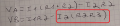# How to solve equations simultaneously

#### BobTPH

Joined Jun 5, 2013
8,085
What have you been taught that might help? Have you studied linear algebra?

#### ArielBendit11

Joined Jul 30, 2023
5
What have you been taught that might help? Have you studied linear algebra?
Man, I only need an example and answer.

#### BobTPH

Joined Jun 5, 2013
8,085
We do not do your homework you. Show us what you have done so far and why you are stuck. If you have studied linear algebra, this should be easy.

#### wayneh

Joined Sep 9, 2010
17,469
See if you gain anything by adding or subtracting the two equations, or multiples of one. Often you can make a term disappear and simplify.

#### MrChips

Joined Oct 2, 2009
29,825
Solving for two variables in two equations is relatively easy.
Solving multi-dimensional equations is much more difficult.
What do you know about matrix mathematics?

#### RoofSheep

Joined Mar 7, 2023
36

#### WBahn

Joined Mar 31, 2012
29,494
View attachment 299430

Hey, this is the first time I deal with this exercise. May someone explain me how to solve it?
Start by asking if the equations even make sense.All of the terms in both equations, except the one circled in red, have units of voltage. The circled term has units of voltage times resistance, which cannot be added to the other terms in that equation.

So there's no point going any further until that is resolved, because you are guaranteed to arrive at a worthless answer.

•wayneh

#### MrAl

Joined Jun 17, 2014
10,898
View attachment 299430

Hey, this is the first time I deal with this exercise. May someone explain me how to solve it?
Hello there,

A quick note here.
Your R2R3 is probably R2+R3 but you better check that as the previous post pointed out.

Once you get that resolved, what you can do is multiply one equation by a constant such that when you subtract the two equations, you effectively get rid of that variable.
For example, say you have one question with this in it:
6*x
and the other equation has this:
2*x

To get rid of 'x' in the first solution, you can multiply that second equation by 3 and that will give you this:
6x in the first and
6x in the second.

Now when you subtract, you get 6x-6x=0, and then you also have that new equation with only one unknown variable which i assume you know how to solve.
Once you find that variable, you can go back and substitute it into one of the equations and then you can solve for that second variable with ease because that's the only variable left to solve for.

#### WBahn

Joined Mar 31, 2012
29,494
Where are these equations coming from?

They are all-but gibberish.Your first equation reduces immediately to V_A = I1·R1 because the last two terms cancel out.

Your two equations for V_A are inconsistent with each other.

•